To provide convenient access to epidemiological data on the coronavirus outbreak, we developed an R package, nCov2019 (https://github.com/GuangchuangYu/nCov2019). Besides detailed real-time statistics, it also includes historical data in China, down to the city-level. We also developed a website (http://www.bcloud.org/e/) with interactive plots and simple time-series forecasts. These analytics tools could be useful in informing the public and studying how this and similar viruses spread in populous countries.

# Installation

To start off, users could utilize the ‘remotes’ package to install it directly from GitHub by running the following in R:

``````library('remotes')
remotes::install_github("GuangchuangYu/nCov2019", dependencies = TRUE)``````

# Query the latest data

To query the latest data, you can load it in with `get_nCov2019()`. By default, the language setting is automatically set to Chinese or English based on the user’s system environment. Of course, users can also use parameter `lang = 'zh'` or `lang = 'en'` to set it explicitly.

Since most of confirmed cases concentrated in China, researchers may more concern about the details in China. So, print the object `x`, you could get the total number of confirmed cases in China.

``````library('nCov2019')
x <- get_nCov2019(lang = 'en')
x ``````
``````China (total confirmed cases): 81385
last update: 2020-03-20 17:24:49 ``````

And then you could use `summary(x)` to get recent Chinese data.

``head(summary(x))``
``````  confirm suspect dead heal nowConfirm nowSevere importedCase deadRate
1      41       0    1    0          0         0            0      2.4
2      41       0    1    0          0         0            0      2.4
3      41       0    2    5          0         0            0      4.9
4      45       0    2    8          0         0            0      4.4
5      62       0    2   12          0         0            0      3.2
6     198       0    3   17          0         0            0      1.5
healRate  date
1      0.0 01.13
2      0.0 01.14
3     12.2 01.15
4     17.8 01.16
5     19.4 01.17
6      8.6 01.18``````

While no region is specified, `x[]` will return the provincial level outbreak statistics in China.

``head(x[]) ``
``````       name confirm suspect dead deadRate showRate  heal healRate showHeal
1     Hubei   67800       0 3132     4.62    FALSE 58381    86.11     TRUE
2 Guangdong    1395       0    8     0.57    FALSE  1322    94.77     TRUE
3     Henan    1273       0   22     1.73    FALSE  1250    98.19     TRUE
4  Zhejiang    1234       0    1     0.08    FALSE  1219    98.78     TRUE
5     Hunan    1018       0    4     0.39    FALSE  1014    99.61     TRUE
6     Anhui     990       0    6     0.61    FALSE   984    99.39     TRUE``````

To obtain a more granular scale data, you only need to specify the province name. For example, to obtain data in Hubei Province.

``head(x['Hubei'])``
``````       name confirm suspect dead deadRate showRate  heal healRate showHeal
1     Wuhan   50005       0 2498     5.00    FALSE 41389    82.77     TRUE
2   Xiaogan    3518       0  128     3.64    FALSE  3349    95.20     TRUE
3 Huanggang    2907       0  125     4.30    FALSE  2782    95.70     TRUE
4  Jingzhou    1580       0   50     3.16    FALSE  1517    96.01     TRUE
5     Ezhou    1394       0   58     4.16    FALSE  1303    93.47     TRUE
6   Suizhou    1307       0   45     3.44    FALSE  1236    94.57     TRUE``````

In addition, by using the argument `by = 'today'`, the number of newly added cases will be return.

``head(x['Hubei', by = 'today'])``
``````       name confirm confirmCuts isUpdated
1     Wuhan       0           0      TRUE
2   Xiaogan       0           0      TRUE
3 Huanggang       0           0      TRUE
4  Jingzhou       0           0      TRUE
5     Ezhou       0           0     FALSE
6   Suizhou       0           0      TRUE``````

Getting global data is also easy, by using `x['global']`, the data frame for the global landscape view of each country will be returned.

``head(x['global'])``
``````           name confirm suspect dead deadRate showRate  heal healRate
1         China   81385     104 3253     4.00    FALSE 71290    87.60
2         Italy   41035       0 3405      8.3    FALSE  4440    10.82
3         Spain   19980       0 1002     5.02    FALSE  1081     5.41
4          Iran   19644       0 1433     7.29    FALSE  6745    34.34
5       Germany   15320       0   44     0.29    FALSE   115     0.75
6 United States   14365       0  212     1.48    FALSE   125     0.87
showHeal
1     TRUE
2    FALSE
3    FALSE
4    FALSE
5    FALSE
6    FALSE``````

If you wanted to visualize the cumulative summary data, an example plot could be the following:

``d <- summary(x)``
``````library(ggplot2)

ggplot(d,
aes(as.Date(date, "%m.%d"), as.numeric(confirm))) +
geom_col(fill = 'firebrick') +
theme_minimal(base_size = 14) +
xlab(NULL) + ylab(NULL) +
scale_x_date(date_labels = "%Y/%m/%d") +
labs(caption = paste("accessed date:", time(x)))``````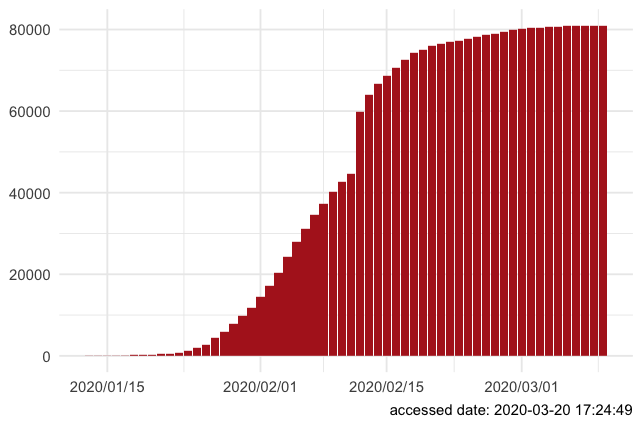And the bar-plot of the latest confirmed diagnosis in Anhui province could be plotted as follow:

``````library(ggplot2)
d = x['Anhui', ] # you can replace Anhui with any province
d = d[order(d\$confirm), ]
ggplot(d, aes(name, as.numeric(confirm))) +
geom_col(fill = 'firebrick') +
theme_minimal(base_size = 14) +
xlab(NULL) + ylab(NULL) +
labs(caption = paste("accessed date:", time(x))) +
scale_x_discrete(limits = d\$name) + coord_flip()``````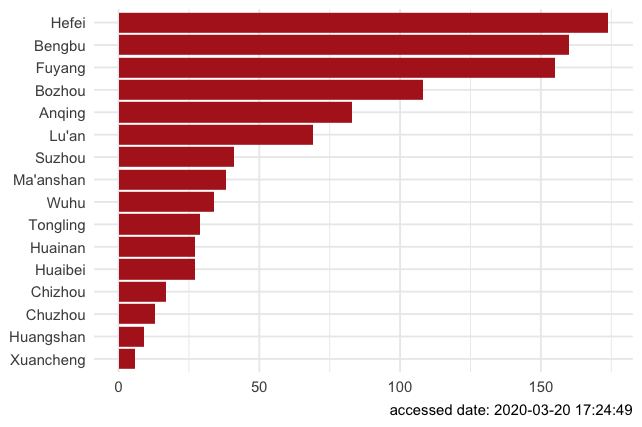# Access detailed historical data

The method for accessing historical data is basically the same as getting the latest data, but entry function is `load_nCov2019()`.

``````library('nCov2019')
y  # this will return update time of historical data``````
``````nCov2019 historical data
last update: 2020-03-19 ``````

For the historical data, currently, we maintain three historical data, one of which is collected and organized from GitHub repo, user will access it by default, or use `load_nCov2019(source = 'github')` to get it.

The second one is obtained from an Chinese website Dingxiangyuan and user could access it by using `load_nCov2019(source = 'dxy')`. And the last one is obtained from the National Health Commission of Chinese, user could get it by using argument `source = 'cnnhc'`. The forms of these data are basically the same, but the default data source has more comprehensive global historical information and also contains older historical data. Users can compare and switch data from different sources.

``````# compare the total confirmed cases in china between data sources
library(nCov2019)
library(ggplot2)
nCov2019_set_country('China')
y = load_nCov2019(lang = 'en', source = 'github')
dxy = load_nCov2019(lang = 'en', source = 'dxy')
nhc = load_nCov2019(lang = 'en', source = 'cnnhc')
dxy_china <- aggregate(cum_confirm ~ + time, summary(dxy), sum)
y_china <- aggregate(cum_confirm ~ + time, summary(y), sum)
nhc_china <- aggregate(cum_confirm ~ + time, summary(nhc), sum)
dxy_china\$source = 'DXY data'
y_china\$source = 'GitHub data'
nhc_china\$source = 'NHC data'
df = rbind(dxy_china, y_china, nhc_china)
ggplot(subset(df, time >= '2020-01-11'),
aes(time,cum_confirm, color = source)) +
geom_line() + scale_x_date(date_labels = "%Y-%m-%d") +
ylab('Confirmed Cases in China') + xlab('Time') + theme_bw() +
theme(axis.text.x = element_text(hjust = 1)) +
theme(legend.position = 'bottom') ``````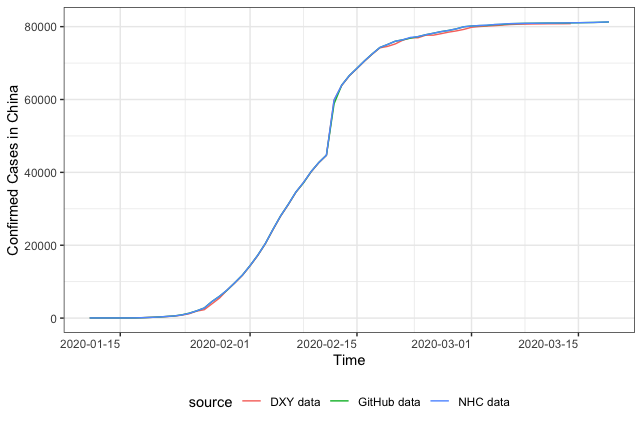Then you can use `summary(y)` to get historical data at the provincial level in China:

``head(summary(y))``
``````        time country province cum_confirm cum_heal cum_dead suspected
1 2019-12-01   China    Hubei           1        0        0         0
2 2019-12-02   China    Hubei           1        0        0         0
3 2019-12-03   China    Hubei           1        0        0         0
4 2019-12-04   China    Hubei           1        0        0         0
5 2019-12-05   China    Hubei           1        0        0         0
6 2019-12-06   China    Hubei           1        0        0         0``````

To get historical data for all cities in China, you can use `y[]` as follow:

``head(y[]) ``
``````        time country province  city cum_confirm cum_heal cum_dead
1 2019-12-01   China    Hubei Wuhan           1        0        0
2 2019-12-02   China    Hubei Wuhan           1        0        0
3 2019-12-03   China    Hubei Wuhan           1        0        0
4 2019-12-04   China    Hubei Wuhan           1        0        0
5 2019-12-05   China    Hubei Wuhan           1        0        0
6 2019-12-06   China    Hubei Wuhan           1        0        0
suspected
1         0
2         0
3         0
4         0
5         0
6         0``````

You can also specify a province name to get the corresponding historical data, for example, extracting historical data from Anhui Province:

``head(y['Anhui'])``
``````          time country province   city cum_confirm cum_heal cum_dead
63  2020-01-21   China    Anhui  Hefei           0        0        0
96  2020-01-22   China    Anhui  Hefei           1        0        0
102 2020-01-22   China    Anhui  Lu'an           0        0        0
198 2020-01-23   China    Anhui  Hefei           6        0        0
199 2020-01-23   China    Anhui Bengbu           1        0        0
200 2020-01-23   China    Anhui Anqing           1        0        0
suspected
63          1
96          3
102         1
198         0
199         0
200         0``````

Similarly, you can get global historical data by specifying the `'global'` parameter.

``````y <- load_nCov2019(lang = 'en', source='github')
d <- y['global']
tail(d)``````
``````           time               country cum_confirm cum_heal cum_dead
3137 2020-03-19 Virgin Islands (U.S.)           2        0        0
3138 2020-03-19               Vietnam          76       16        0
3139 2020-03-19               Mayotte           3        0        0
3140 2020-03-19          South Africa         150        1        0
3141 2020-03-19                Zambia           2        0        0
3142 2020-03-19               Namibia           2        0        0``````

NOTE: The global historical data is not available from source `'dxy'`.

Here are some visualization examples with the historical data.

1. Draw a curve reflecting the number of deaths, confirms, and cures in China.
``````library('tidyr')
library('ggrepel')
library('ggplot2')
d <- subset(y['global'], country == 'China')
d <- gather(d, curve, count, -time, -country)
ggplot(d, aes(time, count, color = curve)) +
geom_point() + geom_line() + xlab(NULL) + ylab(NULL) +
theme_bw() + theme(legend.position = "none") +
geom_text_repel(aes(label = curve),
data = d[d\$time == time(y), ], hjust = 1) +
theme(axis.text.x = element_text(angle = 15, hjust = 1)) +
scale_x_date(date_labels = "%Y-%m-%d",
limits = c(as.Date("2020-01-15"), as.Date("2020-03-20"))) +
labs(title="Number of deaths, confirms, and cures in China") ``````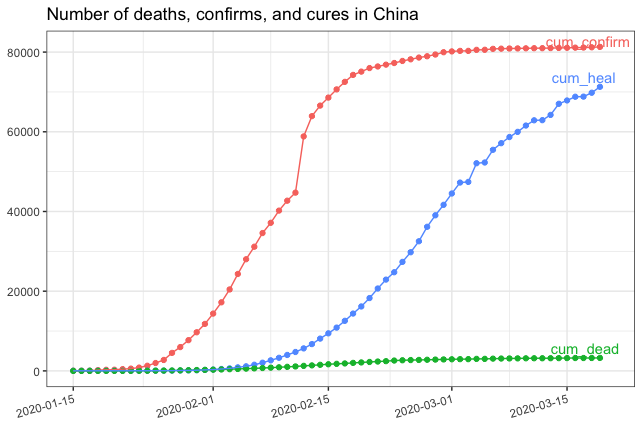1. Outbreak Trend Curves of Top ten Countries Around the World (except China).
``````library('ggrepel')
library('ggplot2')
df <- y['global']
d <- subset(df,country != 'China' & time == time(y))
t10 <- d[order(d\$cum_confirm,decreasing = T),]\$country[1:10]
df <- df[which(df\$country %in% t10),]

ggplot(df, aes(time, as.numeric(cum_confirm),
group = country, color = country)) +
geom_point() + geom_line() +
geom_label_repel(aes(label = country),
data = df[df\$time == time(y), ], hjust = 1) +
theme_bw() + theme(legend.position = 'none') +
xlab(NULL) + ylab(NULL) +
scale_x_date(date_labels = "%Y-%m-%d",
limits = c(as.Date("2020-02-01"), as.Date("2020-03-19"))) +
theme(axis.text.x = element_text(angle = 15, hjust = 1)) +
labs(title = "Outbreak Trend Curves of Top 10 Countries Around the World \n (except China)")``````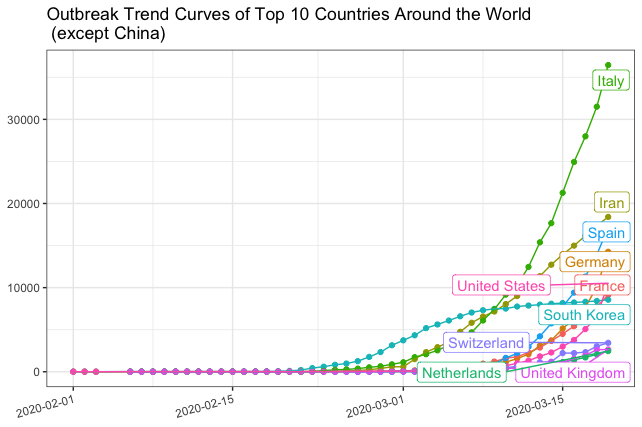1. Growth curve of confirms in Anhui Province, China.
``````y <- load_nCov2019(lang = 'en')
d <- y['Anhui']
ggplot(d, aes(time, as.numeric(cum_confirm),
group = city, color = city)) +
geom_point() + geom_line() +
geom_label_repel(aes(label = city),
data = d[d\$time == time(y), ], hjust = 1) +
theme_minimal(base_size = 14) + theme(legend.position = 'none') +
scale_x_date(date_labels = "%Y-%m-%d") + xlab(NULL) + ylab(NULL) +
theme(axis.text.x = element_text(hjust = 1)) +
labs(title = "Growth curve of confirms in Anhui Province, China")``````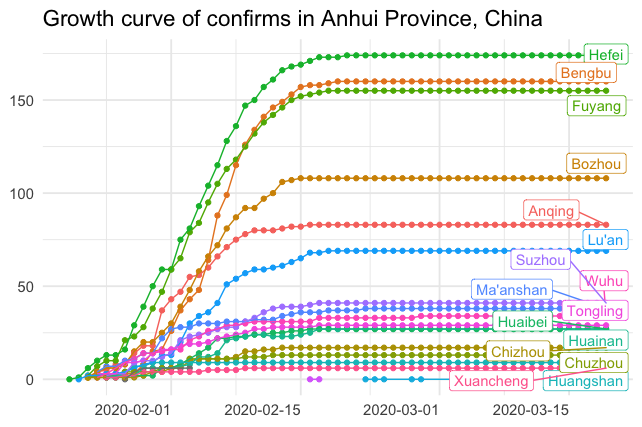1. A heatmap of epidemic situation around the world in the last 7 days.
``````library(ggplot2)
d <- y['global']
max_time <- max(d\$time)
min_time <- max_time - 7
d <- na.omit(d[d\$time >= min_time & d\$time <= max_time,])
dd <- d[d\$time == max(d\$time, na.rm = TRUE),]
d\$country <- factor(d\$country,
levels=unique(dd\$country[order(dd\$cum_confirm)]))
breaks = c(10, 100, 1000, 10000)
ggplot(d, aes(time, country)) +
geom_tile(aes(fill = cum_confirm), color = 'black') +
scale_fill_viridis_c(trans = 'log', breaks = breaks,
labels = breaks) +
xlab(NULL) + ylab(NULL) +
scale_x_date(date_labels = "%Y-%m-%d") + theme_minimal()``````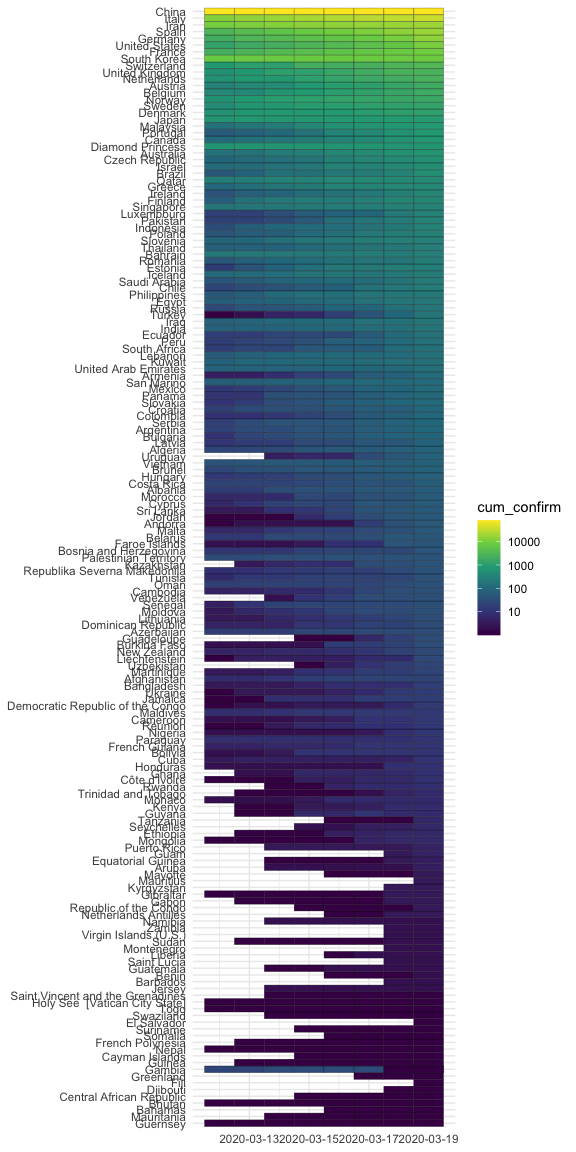The user could get province level data beside China, we current have collected province level information in China, South Korea, United States, Japan, Iran, Italy, Germany and United Kingdom. To get the detail of any country of them, you only need to set the country env as follow:

``````nCov2019_set_country('Italy')
y <- load_nCov2019(lang = 'en', source = 'github')
head(y['province']) # This will return province data of Italy``````
``````           time country         province cum_confirm cum_heal cum_dead
2823 2020-03-15   Italy         Lombardy       13272     2011     1218
2824 2020-03-15   Italy Emilia - Romagna        3093       68      284
2825 2020-03-15   Italy           Veneto        2246      120       63
2826 2020-03-15   Italy           Marche        1133        0       46
2827 2020-03-15   Italy         Piedmont        1111        0       81
2828 2020-03-15   Italy          Tuscany         781       10        8
suspected
2823        NA
2824        NA
2825        NA
2826        NA
2827        NA
2828        NA``````
1. Windrose plot of global confirm cases
``````require(nCov2019)
y <- load_nCov2019(lang = 'en', source='github')
d = y['global']

require(dplyr)
dd <- filter(d, time == time(y)) %>%
arrange(desc(cum_confirm))

dd = dd[1:40, ]
dd\$country = factor(dd\$country, levels=dd\$country)

dd\$angle = 1:40 * 360/40
require(ggplot2)
p <- ggplot(dd, aes(country, cum_confirm, fill=cum_confirm)) +
geom_col(width=1, color='grey90') +
geom_col(aes(y=I(5)), width=1, fill='grey90', alpha = .2) +
geom_col(aes(y=I(3)), width=1, fill='grey90', alpha = .2) +
geom_col(aes(y=I(2)), width=1, fill = "white") +
scale_y_log10() +
scale_fill_gradientn(colors=c("darkgreen", "green", "orange", "firebrick","red"), trans="log") +
geom_text(aes(label=paste(country, cum_confirm, sep="\n"),
y = cum_confirm *.8, angle=angle),
data=function(d) d[d\$cum_confirm > 700,],
size=3, color = "white", fontface="bold", vjust=1)  +
geom_text(aes(label=paste0(cum_confirm, " cases ", country),
y = max(cum_confirm) * 2, angle=angle+90),
data=function(d) d[d\$cum_confirm < 700,],
size=3, vjust=0) +
coord_polar(direction=-1) +
theme_void() +
theme(legend.position="none") +
ggtitle("COVID19 global trend", time(y))``````
``print(p)``